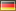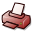# Uncertainty-Aware Numerical Solutions of ODEs by Bayesian Filtering

## DSpace Repository

 Dateien:

 URI: Dokumentart: Dissertation Date: 2021-03-11 Language: English Faculty: 7 Mathematisch-Naturwissenschaftliche Fakultät Department: Informatik Advisor: Hennig, Philipp (Prof. Dr.) Day of Oral Examination: 2020-12-17 DDC Classifikation: 004 - Data processing and computer science510 - Mathematics Keywords: Maschinelles Lernen , Gewöhnliche Differentialgleichung Other Keywords:Probabilistische NumerikProbabilistic NumericsMachine LearningOrdinary Differential Equations License: Order a printed copy:Print-on-Demand Show full item record

### Abstract:

Numerical analysis is the branch of mathematics that studies algorithms that compute approximations of well-defined, but analytically-unknown mathematical quantities. Statistical inference, on the other hand, studies which judgments can be made on unknown parameters in a statistical model. By interpreting the unknown quantity of interest as a parameter and providing a statistical model that relates it to the available numerical information (the `data'), we can thus recast any problem of numerical approximation as statistical inference. In this way, the field of probabilistic numerics introduces new 'uncertainty-aware' numerical algorithms that capture all relevant sources of uncertainty (including all numerical approximation errors) by probability distributions. While such recasts have been a decades-long success story for global optimization and quadrature (under the names of Bayesian optimization and Bayesian quadrature), the equally important numerical task of solving ordinary differential equations (ODEs) has been, until recently, largely ignored. With this dissertation, we aim to further shed light on this area of previous ignorance in three ways: Firstly, we present a first rigorous Bayesian model for initial value problems (IVPs) as statistical inference, namely as a stochastic filtering problem, which unlocks the employment of all Bayesian filters (and smoothers) to IVPs. Secondly, we theoretically analyze the properties of these new ODE filters, with a special emphasis on the convergence rates of Gaussian (Kalman) ODE filters with integrated Brownian motion prior, and explore their potential for (active) uncertainty quantification. And, thirdly, we demonstrate how employing these ODE filters as a forward simulator engenders new ODE inverse problem solvers that outperform classical 'uncertainty-unaware' ('likelihood-free') approaches. This core content is presented in Chapter 2. It is preceded by a concise introduction in Chapter 1 which conveys the necessary concepts and locates our work in the research environment of probabilistic numerics. The final Chapter 3 concludes with an in-depth discussion of our results and their implications.